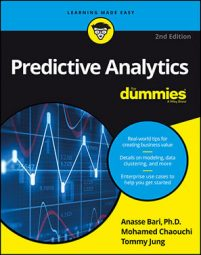##### Predictive Analytics For DummiesOne clustering algorithm offered in `scikit-learn` that can be used in predictive analytics is the mean shift algorithm. This algorithm, like DBSCAN, doesn't require you to specify the number of clusters, or any other parameters, when you create the model. The primary tuning parameter for this algorithm is called the `bandwidth` parameter. You can think of `bandwidth` like choosing the size of a round window that can encompass the data points in a cluster. Choosing a value for bandwidth isn't trivial, so go with the default.

## Running the full dataset

The steps to create a new model with a different algorithm is essentially the same each time:
1. Open a new Python interactive shell session. Use a new Python session so that memory is clear and you have a clean slate to work with.
2. Paste the following code in the prompt and observe the output: ```>>> from sklearn.datasets import load_iris ````>>> iris = load_iris()`
3. Create an instance of mean shift. Type the following code into the interpreter: ```>>> from sklearn.cluster import MeanShift ``````>>> ms = MeanShift() ```Mean shift created with default value for bandwidth.
4. Check which parameters were used by typing the following code into the interpreter: ```>>> ms ````MeanShift(bandwidth=None, bin_seeding=False, cluster_all=True, min_bin_freq=1, n_jobs=1, seeds= None)`
5. Fit the Iris data into the mean shift clustering algorithm by typing the following code into the interpreter: ```>>> ms.fit(iris.data) ```To check the outcome, type the following code into the interpreter:
`>>> ms.labels_`

`array([1, 1, 1, 1, 1, 1, 1, 1, 1, 1, 1, 1, 1, 1, 1, 1, 1,`

`1, 1, 1, 1, 1, 1, 1, 1, 1, 1, 1, 1, 1, 1, 1, 1, 1,`

`1, 1, 1, 1, 1, 1, 1, 1, 1, 1, 1, 1, 1, 1, 1, 1, 0,`

`0, 0, 0, 0, 0, 0, 0, 0, 0, 0, 0, 0, 0, 0, 0, 0, 0,`

`0, 0, 0, 0, 0, 0, 0, 0, 0, 0, 0, 0, 0, 0, 0, 0, 0,`

`0, 0, 0, 0, 0, 0, 0, 0, 0, 0, 0, 0, 0, 1, 0, 0, 0,`

`0, 0, 0, 0, 0, 0, 0, 0, 0, 0, 0, 0, 0, 0, 0, 0, 0,`

`0, 0, 0, 0, 0, 0, 0, 0, 0, 0, 0, 0, 0, 0, 0, 0, 0,`

`0, 0, 0, 0, 0, 0, 0, 0, 0, 0, 0, 0, 0, 0])`

Mean shift produced two clusters (0 and 1).

## Visualizing the clusters

A scatter plot is a good way to visualize the relationship between a large number of data points. It's useful for visually identifying clusters of data and finding data points that are distant from formed clusters.

Let's produce a scatter plot of the DBSCAN output. Type the following code:

`>>> import pylab as pl`

`>>> from sklearn.decomposition import PCA`

`>>> pca = PCA(n_components=2).fit(iris.data)`

`>>> pca_2d = pca.transform(iris.data)`

`>>> pl.figure('Figure 13-7')`

`>>> for i in range(0, pca_2d.shape):`

`>>> if ms.labels_[i] == 1:`

`>>> c1 = pl.scatter(pca_2d[i,0],pca_2d[i,1],c='r',`

`marker='+')`

`>>> elif ms.labels_[i] == 0:`

`>>> c2 = pl.scatter(pca_2d[i,0],pca_2d[i,1],c='g',`

`marker='o')`

`>>> pl.legend([c1, c2], ['Cluster 1', 'Cluster 2')]`

`>>> pl.title('Mean shift finds 2 clusters)`

`>> pl.show()`

The scatter plot output of this code is shown here.

Mean shift found two clusters. You can try to tune the model with the `bandwidth` parameter to see if you can get a three-cluster solution. Mean shift is very sensitive to the `bandwidth` parameter:

• If the chosen value is too big, then the clusters will tend to combine and the final output will be a smaller number of clusters than desired.
• If the chosen value is too small, then the algorithm may produce too many clusters and it will take longer to run.

## Evaluating the model

Mean shift didn't produce the ideal results with the default parameters for the Iris dataset, but a two-cluster solution is in line with other clustering algorithms. Each project has to be examined individually to see how well the number of cluster fits the business problem.

The obvious benefit of using mean shift is that you don’t have to predetermine the number of clusters. In fact, you can use mean shift as a tool to find the number of clusters for creating a K-means model. Mean shift is often used for computer vision applications because it's good at lower dimensions, accommodates clusters of any shape, and accommodates clusters of any size.